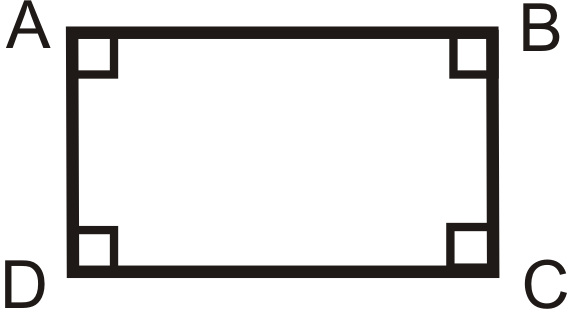# 5.8: Parallelogram Classification

•• Contributed by CK12
• CK12

Rectangles, rhombuses, and squares are parallelograms defined by their diagonals, angles, and sides.

## Classifying Parallelograms

Rectangles, rhombuses (also called rhombi) and squares are all more specific versions of parallelograms, also called special parallelograms.

• A quadrilateral is a rectangle if and only if it has four right (congruent) angles.Figure $$\PageIndex{1}$$

$$ABCD$$ is a rectangle if and only if $$\angle A\cong \angle B\cong \angle C\cong \angle D$$.

• A quadrilateral is a rhombus if and only if it has four congruent sides.Figure $$\PageIndex{2}$$

$$ABCD$$ is a rhombus if and only if $$\overline{AB}\cong \overline{BC} \cong \overline{CD} \cong \overline{AD}$$.

• A quadrilateral is a square if and only if it has four right angles and four congruent sides. By definition, a square is a rectangle and a rhombus.Figure $$\PageIndex{3}$$

$$ABCD$$ is a square if and only if $$\angle A\cong \angle B\cong \angle C\cong \angle D$$ and $$\overline{AB}\cong \overline{BC} \cong \overline{CD} \cong \overline{AD}$$.

You can always show that a parallelogram is a rectangle, rhombus, or square by using the definitions of these shapes. There are some additional ways to prove parallelograms are rectangles and rhombuses, shown below:

1. A parallelogram is a rectangle if the diagonals are congruent.Figure $$\PageIndex{4}$$

$$ABCD$$ is parallelogram. If $$\overline{AC}\cong \overline{BD}$$, then $$ABCD$$ is also a rectangle.

2. A parallelogram is a rhombus if the diagonals are perpendicular.Figure $$\PageIndex{5}$$

$$ABCD$$ is a parallelogram. If $$\overline{AC}\perp \overline{BD}$$, then $$ABCD$$ is also a rhombus.

3. A parallelogram is a rhombus if the diagonals bisect each angle.Figure $$\PageIndex{6}$$

$$ABCD$$ is a parallelogram. If $$\overline{AC}$$ bisects $$\angle BAD$$ and $$\angle BCD$$ and $$\overline{BD}$$ bisects $$\angle ABC$$ and $$\angle ADC$$, then $$ABCD$$ is also a rhombus.

What if you were given a parallelogram and information about its diagonals? How could you use that information to classify the parallelogram as a rectangle, rhombus, and/or square?

Example $$\PageIndex{1}$$

Is a rectangle SOMETIMES, ALWAYS, or NEVER a parallelogram? Explain why.

Solution

A rectangle has two sets of parallel sides, so it is ALWAYS a parallelogram.

Example $$\PageIndex{2}$$

Is a quadrilateral SOMETIMES, ALWAYS, or NEVER a pentagon? Explain why.

Solution

A quadrilateral has four sides, so it will NEVER be a pentagon with five sides.

Example $$\PageIndex{3}$$

What typed of parallelogram are the figures below?Figure $$\PageIndex{7}$$Figure $$\PageIndex{8}$$

Solution

For the first figure, all sides are congruent and one angle is $$135^{\circ}$$, so the angles are not congruent. This is a rhombus.

For the second figure, all four angles are congruent but the sides are not. This is a rectangle.

Example $$\PageIndex{4}$$

Is a rhombus SOMETIMES, ALWAYS, or NEVER a square? Explain why.

Solution

A rhombus has four congruent sides and a square has four congruent sides and angles. Therefore, a rhombus is a square when it has congruent angles. This means a rhombus is SOMETIMES a square.

Example $$\PageIndex{5}$$

List everything you know about the square $$SQRE$$.Figure $$\PageIndex{9}$$

Solution

A square has all the properties of a parallelogram, rectangle and rhombus.

Properties of a Parallelogram Properties of a Rhombus Properties of a Rectangle
• $$\overline{SQ}\parallel \overline{ER}$$
• $$\overline{SQ}\cong \overline{ER}\cong \overline{SE}\cong \overline{QR}$$
• $$m\angle SER=m\angle SQR=m\angle QSE=m\angle QRE=90^{\circ}$$
• $$\overline{SE}\parallel \overline{QR}$$
• $$\overline{SR}\perp \overline{QE}$$
• $$\angle SEQ\cong \angle QER\cong \angle SQE\cong \angle EQR$$
• $$\overline{SR}\cong \overline{QE}$$
• $$\angle QSR\cong \angle RSE\cong \angle QRS\cong \angle SRE$$
• $$\overline{SA}\cong \overline{AR}\cong \overline{QA}\cong \overline{AE}$$

All the bisected angles are $$45^{\circ}$$.

## Review

1. $$RACE$$ is a rectangle. Find:
1. $$RG$$
2. $$AE$$
3. $$AC$$
4. $$EC$$
5. $$m\angle RAC$$Figure $$\PageIndex{10}$$
2. $$DIAM$$ is a rhombus. Find:
1. $$MA$$
2. $$MI$$
3. $$DA$$
4. $$m\angle DIA$$
5. $$m\angle MOA$$Figure $$\PageIndex{11}$$
3. $$CUBE$$ is a square. Find:
1. $$m\angle UCE$$
2. $$m\angle EYB$$
3. $$m\angle UBY$$
4. $$m\angle UEB$$Figure $$\PageIndex{12}$$

For questions 4-15, determine if the quadrilateral is a parallelogram, rectangle, rhombus, square or none.

1.Figure $$\PageIndex{13}$$
2.Figure $$\PageIndex{14}$$
3.Figure $$\PageIndex{15}$$
4.Figure $$\PageIndex{16}$$
5.Figure $$\PageIndex{17}$$
6.Figure $$\PageIndex{18}$$
7.Figure $$\PageIndex{19}$$
8.Figure $$\PageIndex{20}$$
9.Figure $$\PageIndex{21}$$
10.Figure $$\PageIndex{22}$$
11.Figure $$\PageIndex{23}$$
12.Figure $$\PageIndex{24}$$

For questions 16-19 determine if the following are ALWAYS, SOMETIME, or NEVER true. Explain your reasoning.

1. A rectangle is a rhombus.
2. A square is a parallelogram.
3. A parallelogram is regular.
4. A square is a rectangle.

## Vocabulary

Term Definition
rectangle A parallelogram is a rectangle if and only if it has four right (congruent) angles
rhombus A parallelogram is a rhombus if and only if it has four congruent sides
square A parallelogram is a square if and only if it has four right angles and four congruent sides.
converse If a conditional statement is $$p\rightarrow q$$ (if $$p$$, then $$q$$), then the converse is $$q\rightarrow p$$ (if $$q$$, then $$p$$. Note that the converse of a statement is not true just because the original statement is true.
Parallelogram A parallelogram is a quadrilateral with two pairs of parallel sides.
Reflexive Property of Congruence $$\overline{AB}\cong \overline{AB}$$ or $$\angle B\cong \angle B$$

Interactive Element

Video: Classifying Parallelograms Principles - Basic

Activities: Parallelogram Classification Discussion Questions

Study Aids: Parallelograms Study Guide

Practice: Parallelogram Classification

Real World: Parallelograms